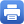Columbia Home
The Many Kinds of Uniformity in Graph Configuration Spaces

Special Seminar

Come join us on Wednesday, January 25, 2023 at 04:30pm in room 520, Professor Eric Ramos (Bowdoin College) will be giving a special lecture titled “The many kinds of uniformity in graph configuration spaces”.

Abstract: For a given topological space X, the (unordered) configuration space of n points on X, F_n(X), is the space of n-element subsets of X. Much of the work on these spaces has considered cases where the underlying space X is a manifold of dimension higher than two. For instance, one famous result of McDuff states that if X is the interior of a compact manifold of dimension at least two with boundary, then for any i the isomorphism class of the homology group H_i(C_n(X)) is independent of n whenever n is big enough. Put more succinctly, if X is a “sufficiently nice” manifold of dimension at least 2, then the configuration spaces C_n(X) exhibit homological stability.

In this talk, we will consider configuration spaces in the cases where X is a graph. That is, when X is 1-dimensional. In this setting we will find that the homology groups H_i(C_n(X)) exhibit extremely regular behaviors in two orthogonal ways. The first, similar to the classical setting, is when X is fixed and n is allowed to grow. In this case we will see that rather than stabilizing, the Betti numbers grow as polynomials in n. The second kind of regular behavior is observed when one fixes n and allows X to vary. In this case we will use extremely powerful structural theorems in graph theory to discover features of the homology groups H_i(C_n(X)) that must be common across all graphs X.

Location: Mathematics Hall, room 520

Date: Wednesday, January 25, 2023 at 04:30pmPrint this page